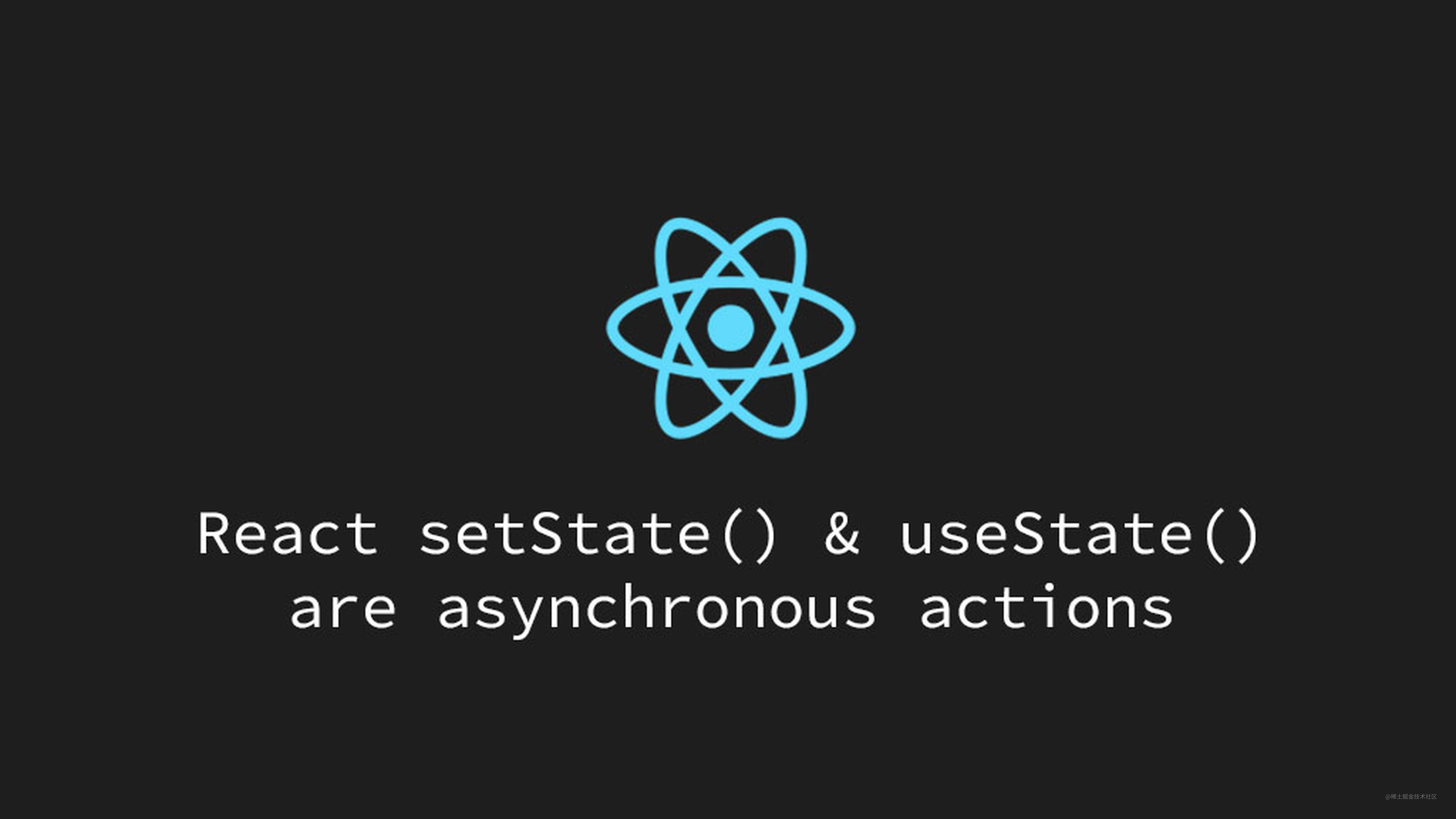# 为什么react的setState是异步的?# 场景

``````import React, { PureComponent } from 'react';

class Demo extends PureComponent {

state = {
count: 0
}

click = () => {
const { count } = this.state;
this.setState({
count: count + 1
});
console.log(this.state.count);

}

render() {
const { count } = this.state;

return (
<div>
<button onClick={this.click}>+1</button>
<div>{count}</div>
</div>
);
}
}

export default Demo;

# 方法一: 回调函数

``````this.setState(
{
count: count + 1
},
() => {
console.log(this.state.count);
}
);

# 方法二: componentDidUpdate

``````import React, { PureComponent } from 'react';

class Demo extends PureComponent {

state = {
count: 0
}

componentDidUpdate() {
const { count } = this.state;
console.log(count);
}

click = () => {
const { count } = this.state;
this.setState({
count: count + 1
});
}

render() {
const { count } = this.state;

return (
<div>
<button onClick={this.click}>+1</button>
<div>{count}</div>
</div>
);
}
}

export default Demo;

# 为何setState是异步的

``````import React, { PureComponent } from 'react';
import Comp1 from '@/components/Comp1';
import Comp2 from '@/components/Comp2';
import Comp3 from '@/components/Comp3';

class Demo extends PureComponent {

state = {
_1: 1,
_2: 2,
_3: 3
}

set1 = () => {
const { _1 } = this.state;
this.setState({
_1: _1 + 1
});
}

set2 = () => {
const { _2 } = this.state;
this.setState({
_2: _2 + 1
});
}

set3 = () => {
const { _3 } = this.state;
this.setState({
_3: _3 + 1
});
}

render() {

return (
<div>
<Comp1 click={this.set1} />
<Comp2 click={this.set2} />
<Comp3 click={this.set3} />
</div>
);
}
}

export default Demo;